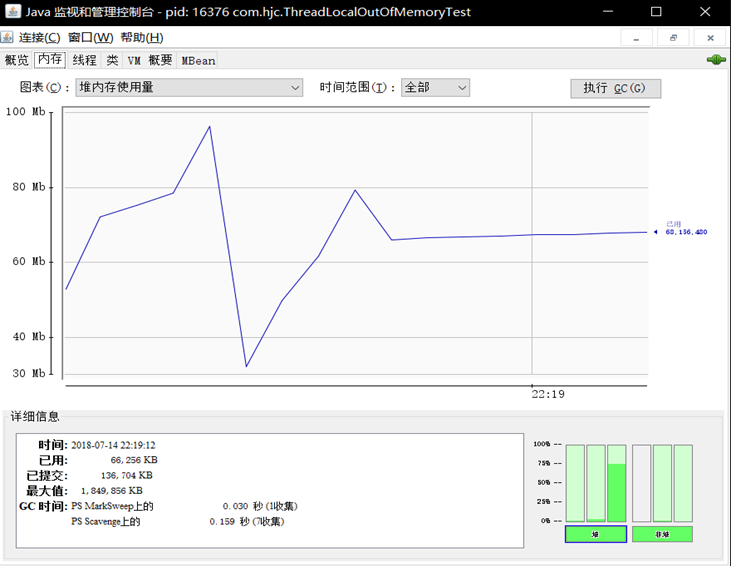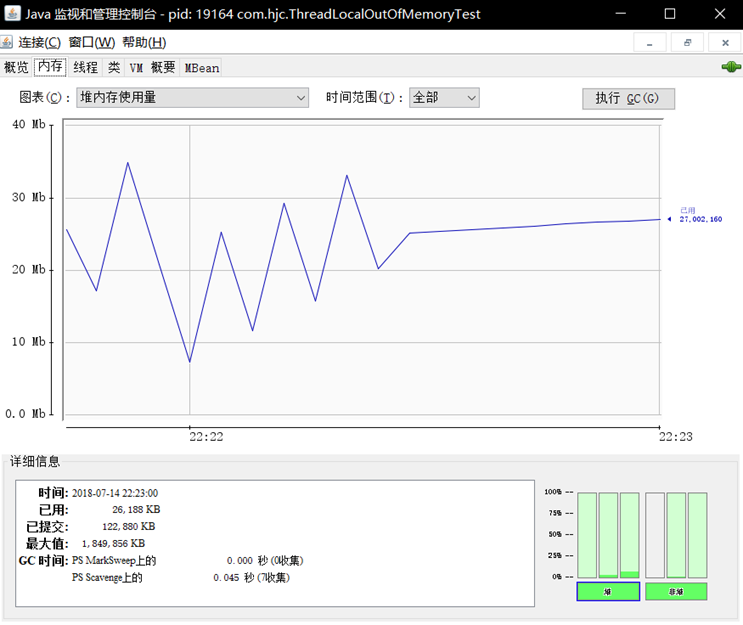/**
* Created by cong on 2018/7/14.
*/
static class LocalVariable {
private Long[] a = new Long[1024*1024];
}

// (1)
// (2)

public static void main(String[] args) throws InterruptedException {
// (3)
for (int i = 0; i < 50; ++i) {
poolExecutor.execute(new Runnable() {
public void run() {
// (4)
localVariable.set(new LocalVariable());
// (5)
System.out.println("use local varaible");
//                    localVariable.remove();

}
});

}
// (6)
System.out.println("pool execute over");
}
}从第一次运行结果可知，当主线程处于休眠时候进程占用了大概 75M 内存，打开 localVariable.remove() 注释后第二次运行则占用了大概 25M 内存，可知 没有写 localVariable.remove() 时候内存发生了泄露，下面分析下泄露的原因，如下：如上图 ThreadLocalMap 内部是一个 Entry 数组, Entry 继承自 WeakReference，Entry 内部的 value 用来存放通过 ThreadLocal 的 set 方法传递的值，那么 ThreadLocal 对象本身存放到哪里了吗?

Entry(ThreadLocal<?> k, Object v) {
super(k);
value = v;
}

public WeakReference(T referent) {
super(referent);
}

Reference(T referent) {
this(referent, null);
}

Reference(T referent, ReferenceQueue<? super T> queue) {
this.referent = referent;
this.queue = (queue == null) ? ReferenceQueue.NULL : queue;
}

private void remove(ThreadLocal<?> key) {

Entry[] tab = table;
int len = tab.length;
int i = key.threadLocalHashCode & (len-1);
//(2)这里使用循环是防止快速定位失效后，变量table数组
for (Entry e = tab[i]; e != null; e = tab[i = nextIndex(i, len)]) {
//(3)找到
if (e.get() == key) {
e.clear();
//(5)清理key为null的元素
expungeStaleEntry(i);
return;
}
}
}
private int expungeStaleEntry(int staleSlot) {
Entry[] tab = table;
int len = tab.length;
//（6）去掉去value的引用
tab[staleSlot].value = null;
tab[staleSlot] = null;
size--;
Entry e;
int i;
for (i = nextIndex(staleSlot, len); (e = tab[i]) != null; i = nextIndex(i, len)) {
//(7)如果key为null,则去掉对value的引用。
if (k == null) {
e.value = null;
tab[i] = null;
size--;
} else {
int h = k.threadLocalHashCode & (len - 1);
if (h != i) {
tab[i] = null;
while (tab[h] != null)
h = nextIndex(h, len);
tab[h] = e;
}
}
}
return i;
}